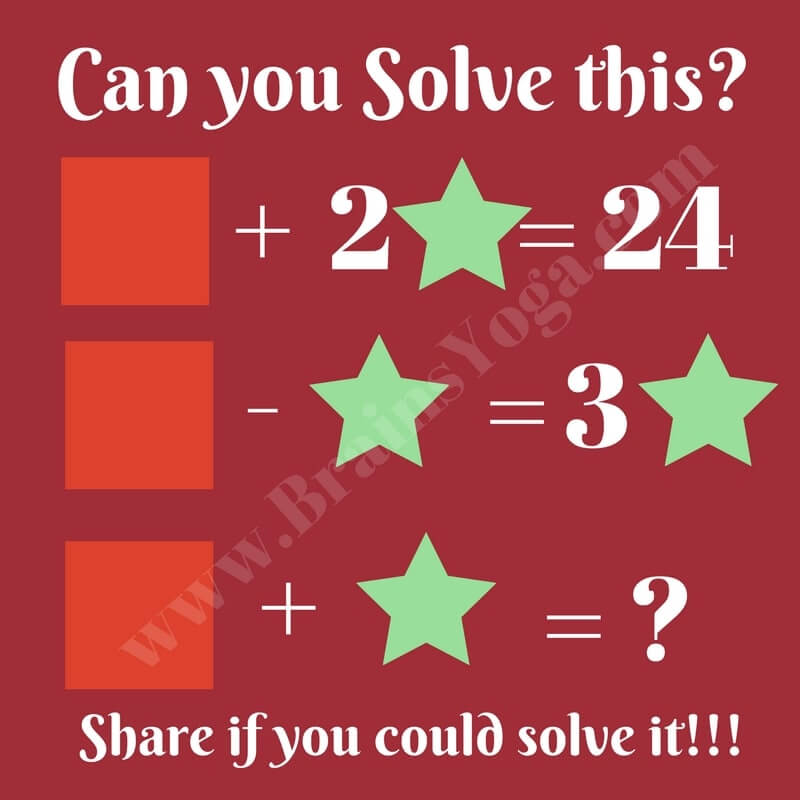This is a fun Maths Brain Teaser for school students. This is an Algebraic puzzle in which your challenge is to find the values of the different variables in the maths equations. Once you are able to find the values of these variables, solve the last equation. Can you solve this Maths Picture Brain Teaser?Maths Picture Brain Teaser